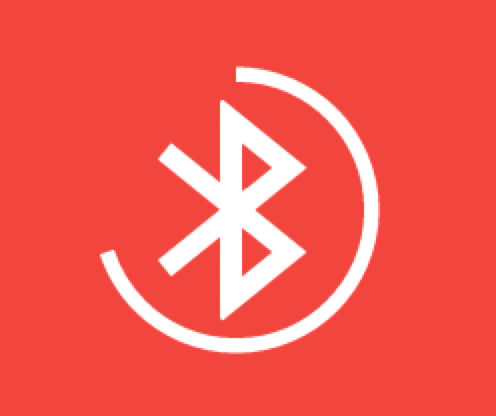In my previous post, I covered the basics of a new type of Android drawable introduced in Lollipop for scalable graphics.

In and by itself this is already a very powerful new addition. But to see what else we can do with this, let’s reuse the vector drawable example from the previous post:

``````<vector xmlns:android="http://schemas.android.com/apk/res/android"
android:height="96dp"
android:width="96dp"
android:viewportHeight="48"
android:viewportWidth="48" >
<group>
<path
android:fillColor="@color/black_primary"
android:pathData="M12 36l17-12-17-12v24zm20-24v24h4V12h-4z" />
</group>
</vector>
``````

I didn’t touch on it at the time but you may have noticed that our `path` element is actually contained inside another element called a `group`.

For the sake of displaying vector drawable, this is not a very interesting element. Now if I paste below the list of other attributes you can set on `<group/>`, you should see something emerging:

Extra group attributes
rotation, scaleX, scaleY, translateX, translateY.

You will have surely recognized those are the same attributes we use to manipulate our `View` instances when animating them.

Enter, Animated Vector Drawable.

Animated Vector Drawables are actually more of a meta-type bridging several other pieces together much like a State List Drawable (and like the later, they also are drawables themselves).

Here is an example of an animated vector drawable that we will use later on (also stored in your `drawable` resource folder):

``````<animated-vector xmlns:android="http://schemas.android.com/apk/res/android"
<target
android:name="circleGroup"
android:animation="@anim/circle_expand" />
<target
android:name="circlePath"
android:animation="@anim/circle_path" />
</animated-vector>
``````

Animated Vector Drawables are declared using the `<animated-vector/>` element. The first thing you need to tie in is which vector drawable the animated version is going to use as a base, this is set in the `android:drawable` attribute at the top level.

The rest of the file contains a bunch of different `<target/>` elements, those are where we are going to set up which animations are ran and on what part of the vector drawable they will be ran.

But first, here is the definition of the `bluetooth_loading_vector` referenced in the animated vector:

``````<?xml version="1.0" encoding="utf-8" ?>
<vector xmlns:android="http://schemas.android.com/apk/res/android"
android:height="64dp"
android:width="64dp"
android:viewportHeight="192"
android:viewportWidth="192" >
<group>
<path
android:fillColor="@color/vector_foreground_color"
android:pathData="M87.3996,113.161L85.3665,112.191L85.3665,23.517L87.3996,22.5479L143.293,67.8542L87.3996,113.161ZM99.9498,84.2151L120.134,67.8542L99.9498,51.4934L99.9498,84.2151Z" />
<path
android:fillColor="@color/vector_foreground_color"
android:pathData="M87.4147,169.459L85.3665,168.484L85.3665,79.6589L87.4147,78.684L143.277,124.071L87.4147,169.459ZM99.9498,140.484L120.15,124.071L99.9498,107.659L99.9498,140.484Z" />
<path
android:fillColor="@color/vector_foreground_color"
android:pathData="M43.8495,129.345L103.262,81.2217L112.186,92.2392L52.7734,140.362L43.8495,129.345Z" />
<path
android:fillColor="@color/vector_foreground_color"
android:pathData="M43.8495,62.2562L103.262,110.379L112.186,99.3616L52.7734,51.2387L43.8495,62.2562Z" />
</group>
<group
android:name="circleGroup"
android:pivotX="96.0"
android:pivotY="96.0"
android:rotation="0">
<path
android:name="circlePath"
android:strokeColor="@color/vector_foreground_color"
android:strokeWidth="16"
android:strokeAlpha="1"
android:trimPathEnd="0.7"
android:pathData="M96,5.56405C145.946,5.56405 186.436,46.0536 186.436,96C186.436,145.946 145.946,186.436 96,186.436C46.0536,186.436 5.56405,145.946 5.56405,96C5.56405,46.0536 46.0536,5.56405 96,5.56405Z" />
</group>
</vector>
``````

And what it looks like when rasterized:This vector drawble is a bit longer than the previous one, but there are two main things to notice:

• The drawable is arranged in two distinct groups
• One group and one path have a name attached, mapping back to the animated vector’s target elements

The way we have layed-out and named our vector drawable means we can now directly reference the relevant parts we want to manipulate.

For instance in our case, we are only interested in the ring around the Bluetooth logo which is why only this piece is actually named in our vector drawable.

To close the loop (so to speak), here are the two animation definition files referenced by the animated vector drawable (placed each in your `anim` resource folder):

``````<!-- Resources\anim\circle_expand.xml -->
<set xmlns:android="http://schemas.android.com/apk/res/android">
<objectAnimator
android:propertyName="scaleX"
android:valueFrom="1.0"
android:valueTo="2.5"
android:valueType="floatType"
android:repeatCount="-1" />
<objectAnimator
android:propertyName="scaleY"
android:valueFrom="1.0"
android:valueTo="2.5"
android:valueType="floatType"
android:repeatCount="-1" />
<objectAnimator
android:propertyName="rotation"
android:valueFrom="0"
android:valueTo="360"
android:valueType="floatType"
android:repeatCount="-1" />
</set>

<!-- Resources\anim\circle_path.xml -->
<set xmlns:android="http://schemas.android.com/apk/res/android">
<objectAnimator
android:propertyName="trimPathEnd"
android:valueFrom="0"
android:valueTo="1"
android:valueType="floatType"
android:repeatCount="-1" />
<objectAnimator
android:propertyName="strokeAlpha"
android:valueFrom="1"
android:valueTo="0"
android:valueType="floatType"
android:repeatCount="-1" />
</set>
``````

Since this is meant as a continous animation, we must ensure that the repeat count of each animator is `-1` (a synonym for ‘infinite’) so that the process runs forever.

In the remaning bits, we are simply animating a bunch of property on both the group (for transformations) and on the path (for the stroke style) like we would on a normal view.

The last thing to do is load the animated vector drawable into a continuously animating View, typically a `ProgressBar`:

``````<FrameLayout
android:layout_width="match_parent"
android:layout_height="wrap_content"
android:background="?android:attr/colorPrimary"
android:clipChildren="false"